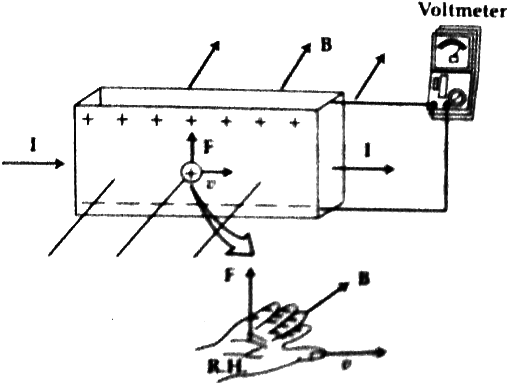# Experiment of Hall’s Effect

Hall’s Effect experiment

If a magnetic field is applied perpendicular to the flow of current, then a potential is created normal to both that of current and the magnetic field. This effect is called Hall Effect and the generated potential is called Hall potential or Hall voltage.

The Hall effect was discovered in 1879 by Edwin Hall while he was working on his doctoral degree at Johns Hopkins University in Baltimore, Maryland. Eighteen years before the electron was discovered, his measurements of the tiny effect produced in the apparatus he used were an experimental tour de force, published under the name “On a New Action of the Magnet on Electric Currents”.

In the figure, the method for the determination of the nature of charges and their numbers are shown. Let us take a thin and wide strip of metallic conductor. Let the current flow along the length of this strip be ‘I’.

Let this strip be placed in a uniform magnetic field B. The strip is placed in such a way as the direction of the magnetic field is perpendicular to the wide surface of the strip. Suppose, the flow of current is due to the flow of positive charges.We know, charges are deflected by magnetic force when a magnetic field is applied on the moving charges. According to Fleming’s right-hand rule, the force F acts upward of the strip (since charges are positive). But if the charges are negative, the reverse phenomenon will happen. That means the force acts downward of this strip. In this experiment, positive charges will accumulate on the upper face of the strip an equal amount of negative charges will accumulate on the lower face of the strip. An electromotive force will be created due to the accumulation of positive and negative charges on the opposite faces of the strip. Higher potential will be created on the upper face and lower potential on the lower face of the strip. This electromotive force or potential difference created between the two faces is called the Hall emf or Hall voltage. Hall voltage can be measured by a voltmeter (shown in the figure). These accumulated positive and negative charges will create an electric field. Now, due to the electric field electric force will act on the flow of current which will be opposite to the magnetic force. An equilibrium is established when these two forces are equal.

Again, if the current is due to the flow of negative charges, then the direction of the generated Hall emf will be opposite.

From this experiment, both the nature of charges as well as the number of charges can be determined.